# vivid

## Introduction

Variable importance, interaction measures and partial dependence plots are important summaries in the interpretation of statistical and machine learning models. In this vignette we describe new visualization techniques for exploring these model summaries. We construct heatmap and graph-based displays showing variable importance and interaction jointly, which are carefully designed to highlight important aspects of the fit. We describe a new matrix-type layout showing all single and bivariate partial dependence plots, and an alternative layout based on graph Eulerians focusing on key subsets. Our new visualisations are model-agnostic and are applicable to regression and classification supervised learning settings. They enhance interpretation even in situations where the number of variables is large and the interaction structure complex. Our R package `vivid` (variable importance and variable interaction displays) provides an implementation.

## Install instructions

Some of the plots used by `vivid` are built upon the `zenplots` package which requires the `graph` package from BioConductor. To install the `graph` and `zenplots` packages use:

`if (!requireNamespace("graph", quietly = TRUE)){`
`install.packages("BiocManager")`
`BiocManager::install("graph")`
`}`
`install.packages("zenplots")`

Now we can install `vivid` by using:

`install.packages("vivid")`

Alternatively you can install the latest development version of the package in R with the commands:

`if(!require(remotes)) install.packages('remotes')`
`remotes::install_github('AlanInglis/vividPackage')`

We then load the required packages. `vivid` to create the visualizations and some other packages to create various model fits.

``````library(vivid) # for visualisations
library(randomForest) # for model fit
library(mlr3)         # for model fit
library(mlr3learners) # for model fit
library(ranger)       # for model fit
library(ggplot2) ``````

# Section 1: Data and model fits

## Data used in this vignette:

The data used in the following examples is simulated from the Friedman benchmark problem 11. This benchmark problem is commonly used for testing purposes. The output is created according to the equation:

\[y = 10 sin(π x_1 x_2) + 20 (x_3 - 0.5)^2 + 10 x_4 + 5 x_5 + e\]

For the following examples we set the number of features to equal 9 and the number of samples is set to 350 and fit a `randomForest` random forest model with \(y\) as the response. As the features \(x_1\) to \(x_5\) are the only variables in the model, therefore \(x_6\) to \(x_{9}\) are noise variables. As can be seen by the above equation, the only interaction is between \(x_1\) and \(x_2\)

Create the data:

``````set.seed(101)
genFriedman <- function(noFeatures = 10,
noSamples = 100,
sigma = 1
) {
# Set Values
n <- noSamples # no of rows
p <- noFeatures # no of variables
e <- rnorm(n, sd = sigma)

# Create matrix of values
xValues <- matrix(runif(n * p, 0, 1), nrow = n) # Create matrix
colnames(xValues) <- paste0("x", 1:p) # Name columns
df <- data.frame(xValues) # Create dataframe

# Equation:
# y = 10sin(πx1x2) + 20(x3−0.5)^2 + 10x4 + 5x5 + ε
y <- (10 * sin(pi * df\$x1 * df\$x2) + 20 * (df\$x3 - 0.5)^2 + 10 * df\$x4 + 5 *    df\$x5 + e)
df\$y <- y
df
}

myData <- genFriedman(noFeatures = 9, noSamples = 350, sigma = 1)``````

## Model fit

Here we create two model fits. We create a random forest fit from the `randomForest` package.

• Create a `randomForest` model:
``````set.seed(100)
rf <- randomForest(y ~ ., data = myData, importance = TRUE)``````

Note that for a `randomForest` model, if `importance = TRUE`, then when running the `vivi` function below an importance type must also be selected (ie., `"%IncMSE"` or `"IncNodePurity"`) via the `importanceType` argument.

## vivi function

To begin, we use the `vivi` function to create a symmetrical matrix filled with pair-wise interaction strengths on the off-diagonals and variable importance on the diagonal. The matrix is ordered so that variables with high interaction strength and importance are pushed to the top left. The `vivi` uses Friedman’s unnormalized H-Statistic to calculate the pair-wise interaction strength and uses either embedded feature selection methods to determine the variable importance, or if the supplied model does not support an embedded variable importance measure an agnostic permutation approach will be applied automatically to generate the importance values. The unnormalized version of the H-statistic was chosen to have a more direct comparison of interaction effects across pairs of variables and the results of H are on the scale of the response.

This function works with multiple model fits and results in a matrix which can be supplied to the plotting functions. The predict function argument uses `condvis2::CVpredict` by default, which works for many fit classes.

Note: For the purposes of speed, the grid size (i.e., `gridSize` - the size of the gid on which the evaluations are made) and the number of rows subsetted (`nmax`) are small. This achieve more accurate results, incerease both the grid size and the number of rows used.

• Create a matrix from the random forest fit to be supplied to the plotting functions.
``````set.seed(101)
rf_fit  <- vivi(fit = rf,
data = myData,
response = "y",
gridSize = 10,
importanceType = "%IncMSE",
nmax = 100,
reorder = TRUE,
class = 1,
predictFun = NULL)``````

# Section 2: Visualizing the results

### Heatmap plot

The first visualization option supplied by `vivid` creates a heatmap plot displaying variable importance on the diagonal and variable interaction on the off-diagonal. As mentioned above, the matrix created by `vivi` is ordered. using a seriation method This will push variables of interest to the top left of the heatmap plot.

``viviHeatmap(mat = rf_fit) + ggtitle("rf heatmap")``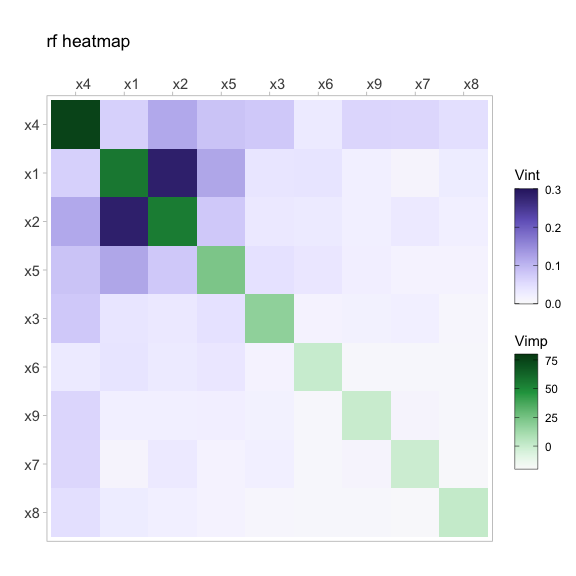Fig 1.0: Heatmap of a random forest fit displaying 2-way interaction strength on the off diagonal and individual variable importance on the diagonal. \(x_1\) and \(x_2\) show a strong interaction with \(x_4\) being the most important for predicting \(y\).

### Network plot

An alternative to the heatmap plot, is a network graph. This has the advantage of allowing the user to quickly identify which variables have a strong interaction in a model. The importance of the variable is represented by both the size of the node (with larger nodes meaning they have greater importance) and the colour of the node. Importance is displayed by using a gradient of white to red, representing the low to high values. The two-way interaction strengths between variables are represented by the connecting lines (or edges). Both the size and colour of the edge are used to highlight interaction strength. Thicker lines between variables indicate a greater interaction strength. The interaction strength values are displayed by using a gradient of white to dark blue, representing the low to high values.

``viviNetwork(mat = rf_fit)``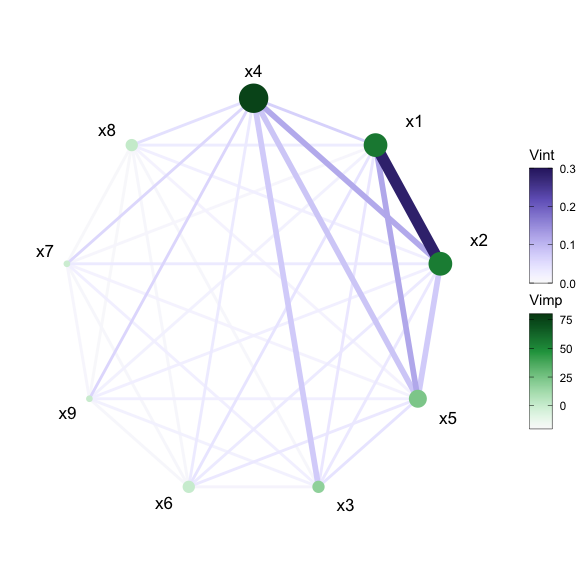Fig 2.0: Network plot of a random forest fit displaying 2-way interaction strength and individual variable importance. \(x_1\) and \(x_2\) show a strong interaction with \(x_4\) being the most important for predicting \(y\).

We can also filter out any interactions below a set value using the `intThreshold` argument. This can be useful when the number of variables included in the model is large or just to highlight the strongest interactions. By default, unconnected nodes are displayed, however, they can be removed by setting the argument `removeNode = T`.

``viviNetwork(mat = rf_fit, intThreshold = 0.12, removeNode = F)``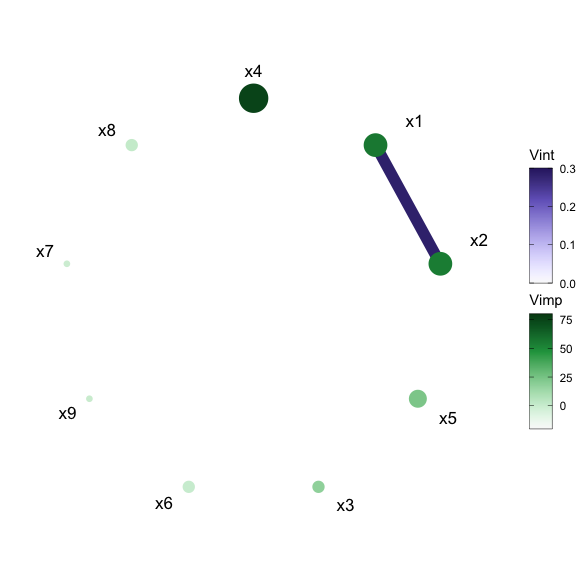Fig 2.2: Filtered Network plot of a random forest fit displaying all nodes with a threshold value of 0.12. At this threshold \(x_1\) and \(x_2\) remain as they have a strong interaction. A very weak interaction can also been seen between \(x_4\) and \(x_1\).
``viviNetwork(mat = rf_fit, intThreshold = 0.12, removeNode = T)``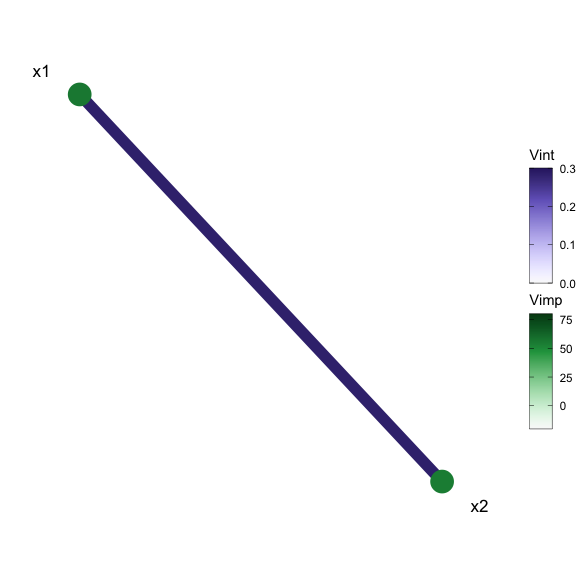Fig 2.3: Filtered Network plot of a random forest fit with unconnected nodes removed and a threshold value of 0.12.

The network plot offers multiple customization possibilities when it comes to displaying the network style plot through use of the `layout` argument. The default layout is a circle but the argument accepts any `igraph` layout function or a numeric matrix with two columns, one row per node.

``````viviNetwork(mat = rf_fit,
layout = cbind(c(1,1,1,1,2,2,2,2,2), c(1,2,4,5,1,2,3,4,5)))``````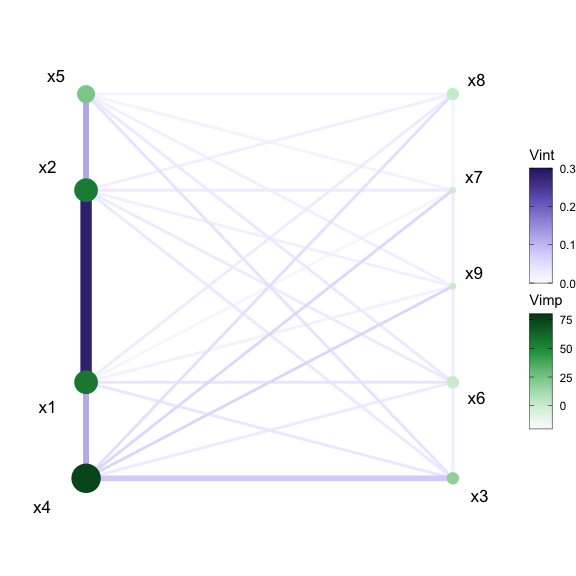Fig 2.4: Network plot of a random forest fit using custom layout.

Finally, for the network plot to highlight any relationships in the model fit, we can cluster variables together using the `cluster` argument. This argument can either accept a vector of cluster memberships for nodes or an igraph clustering function.

``````set.seed(1701)
viviNetwork(mat = rf_fit, cluster = igraph::cluster_fast_greedy)``````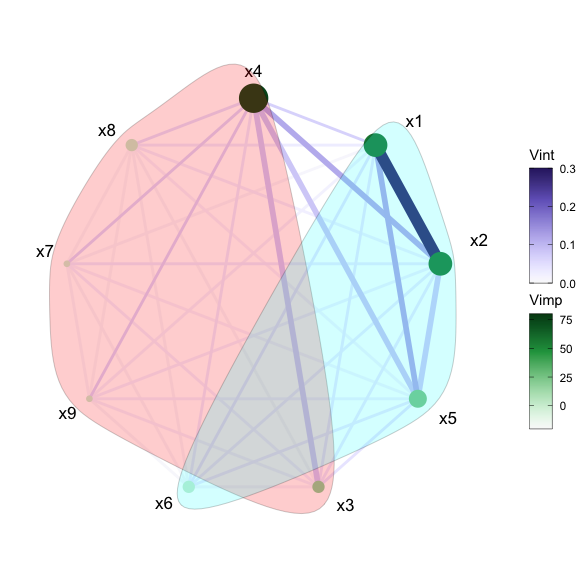Fig 2.5: Clustered network plot of a random forest fit.

The clustered plot in Fig 2.5 shows two clustered groups. As mentioned above, to get more sensible clustered groups, both `gridSize` and `nmax` should be increased.

### Generalized partial dependence pairs plot

This function creates a generalized pairs plot style matrix plot of the 2D partial dependence (PD) of each of the variables in the upper diagonal, the individual partial dependence plots (PDP) and ice curves (ICE) on the diagonal and a scatter-plot of the data on the lower diagonal. The PDP shows the marginal effect that one or two features have on the predicted outcome of a machine learning model2. A partial dependence plot is used to show whether the relationship between the response variable and a feature is linear or more complex. As PD is calculated on a grid, this may result in the PDP extrapolating where there is no data. To solve this issue we calculate a convex hull around the data and remove any points that fall outside the convex hull. This is illustrated in the classification example in Section 3.0. In Fig 3.0 below, we display the generalized partial dependence pairs plot (GPDP) for the random forest fit on the Friedman data.

``````set.seed(1701)
pdpPairs(data = myData, fit = rf, response = "y", nmax = 50, gridSize = 10)
#> Generating ice/pdp fits... waiting...
#> Finished ice/pdp``````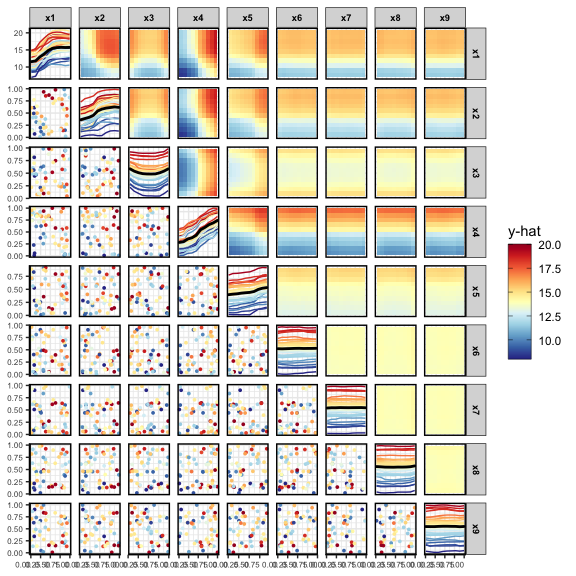Fig 3.0: GPDP of a random forest fit on the Friedman data. From the plot we can see a clear interaction between \(x_1\) and \(x_2\). This can be seen in both the changing ICE curves and 2-way PDPs

As calculating the PD can computationally expensive. To speed the process up we sample the data and by default only display 30 ICE curves per variable on the diagonal (although this cab be changed via function arguments). We can also subset the data to only display a particular set of variables, as shown in Fig 3.1 below.

``````set.seed(1701)
pdpPairs(data = myData, fit =  rf, response = "y", nmax = 50, gridSize = 10,
vars = c("x1", "x2", "x3", "x4", "x5"))
#> Generating ice/pdp fits... waiting...
#> Finished ice/pdp``````